xBäcklund transformEncyclopedia
In mathematics
Mathematics
Mathematics is the study of quantity, space, structure, and change. Mathematicians seek out patterns and formulate new conjectures. Mathematicians resolve the truth or falsity of conjectures by mathematical proofs, which are arguments sufficient to convince other mathematicians of their validity...

, Bäcklund transforms or Bäcklund transformations (called after the Swedish mathematician Albert Victor Bäcklund
Albert Victor Bäcklund
Albert Victor Bäcklund was a Swedish mathematician and physicist. He was a professor at Lund University and its rector from 1907 to 1909....

) relate partial differential equation
Partial differential equation
In mathematics, partial differential equations are a type of differential equation, i.e., a relation involving an unknown function of several independent variables and their partial derivatives with respect to those variables...

s and their solutions. They are an important tool in soliton theory and integrable system
Integrable system
In mathematics and physics, there are various distinct notions that are referred to under the name of integrable systems.In the general theory of differential systems, there is Frobenius integrability, which refers to overdetermined systems. In the classical theory of Hamiltonian dynamical...

s. A Bäcklund transform is typically a system of first order partial differential equations relating two functions, and often depending on an additional parameter. It implies that the two functions separately satisfy partial differential equations, and each of the two functions is then said to be a Bäcklund transformation of the other.

A Bäcklund transform which relates solutions of the same equation is called an invariant Bäcklund transform or auto-Bäcklund transform. If such a transform can be found, much can be deduced about the solutions of the equation especially if the Bäcklund transform contains a parameter. However, no systematic way of finding Bäcklund transforms is known.

## History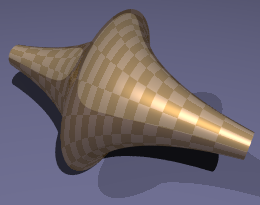Bäcklund transforms have their origins in differential geometry: the first nontrivial example is the transformation of pseudospherical surfaces introduced by L. Bianchi
Luigi Bianchi
- External links :* offers translations of some of Bianchi's papers, plus a biography of Bianchi.* PDF copy at * * * *...

and A.V. Bäcklund
Albert Victor Bäcklund
Albert Victor Bäcklund was a Swedish mathematician and physicist. He was a professor at Lund University and its rector from 1907 to 1909....

in the 1880s. This is a geometrical construction of a new pseudospherical surface from an initial such surface using a solution of a linear differential equation
Linear differential equation
Linear differential equations are of the formwhere the differential operator L is a linear operator, y is the unknown function , and the right hand side ƒ is a given function of the same nature as y...

. Pseudospherical surfaces can be described as solutions of the sine-Gordon equation
Sine-Gordon equation
The sine–Gordon equation is a nonlinear hyperbolic partial differential equation in 1 + 1 dimensions involving the d'Alembert operator and the sine of the unknown function. It was originally considered in the nineteenth century in the course of study of surfaces of constant negative...

, and hence the Bäcklund transformation of surfaces can be viewed as a transformation of solutions of the sine-Gordon equation.

## The Cauchy–Riemann equations

The prototypical example of a Bäcklund transform is the Cauchy–Riemann system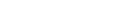which relates the real and imaginary parts u and v of a holomorphic function
Holomorphic function
In mathematics, holomorphic functions are the central objects of study in complex analysis. A holomorphic function is a complex-valued function of one or more complex variables that is complex differentiable in a neighborhood of every point in its domain...

. This first order system of partial differential equations has the following properties.
1. If u and v are solutions of the Cauchy–Riemann equations, then u is a solution of the Laplace equation (i.e., a harmonic function
Harmonic function
In mathematics, mathematical physics and the theory of stochastic processes, a harmonic function is a twice continuously differentiable function f : U → R which satisfies Laplace's equation, i.e....

), and so is v. This follows straightforwardly by differentiating the equations with respect to x and y and using the fact that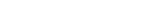2. Conversely if u is a solution of Laplace's equation, then there exist functions v which solve the Cauchy–Riemann equations together with u.

Thus, in this case, a Bäcklund transformation of a harmonic function is just a conjugate harmonic function. The above properties mean, more precisely, that Laplace's equation for u and Laplace's equation for v are the integrability conditions for solving the Cauchy–Riemann equations.

These are the characteristic features of a Bäcklund transform. If we have a partial differential equation in u, and a Bäcklund transform from u to v, we can deduce a partial differential equation satisfied by v.

This example is rather trivial, because all three equations (the equation for u, the equation for v and the Bäcklund transform relating them) are linear. Bäcklund transforms are most interesting when just one of the three equations is linear.

## The sine-Gordon equation

Suppose that u is a solution of the sine-Gordon equation
Sine-Gordon equation
The sine–Gordon equation is a nonlinear hyperbolic partial differential equation in 1 + 1 dimensions involving the d'Alembert operator and the sine of the unknown function. It was originally considered in the nineteenth century in the course of study of surfaces of constant negative...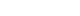Then the system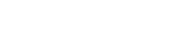where a is an arbitrary parameter, is solvable for a function v which will also satisfy the sine-Gordon equation. This is an example of an auto-Bäcklund transform.

By using a matrix system, it is also possible to find a linear Bäcklund transform for solutions of sine-Gordon equation.

## The Liouville equation

A Bäcklund transform can turn a non-linear partial differential equation into a simpler, linear, partial differential equation.

For example, if u and v are related via the Bäcklund transform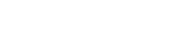where a is an arbitrary parameter, and if u is a solution of the Liouville equation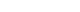then v is a solution of the much simpler equation,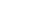, and vice versa.

We can then solve the (non-linear) Liouville equation by working with a much simpler linear equation.
The source of this article is wikipedia, the free encyclopedia.  The text of this article is licensed under the GFDL.